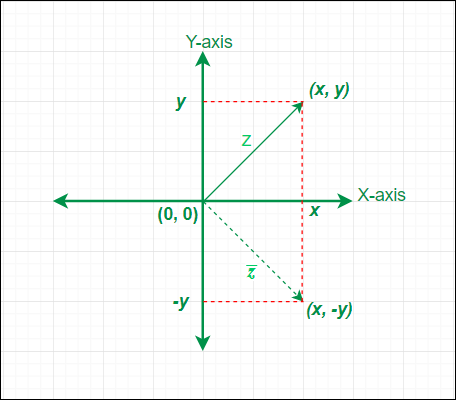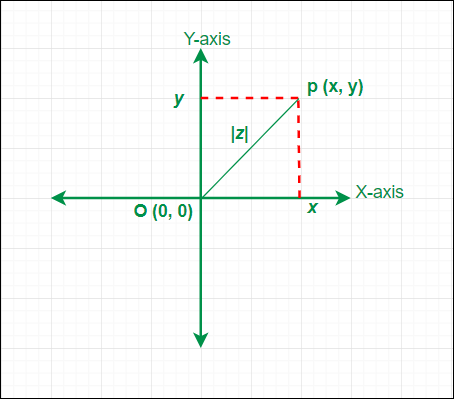Open in App
Not now

# Complex Conjugate

• Difficulty Level : Easy
• Last Updated : 21 Feb, 2021

A complex number is a number represented in the form of (x + i y); where x & y are real numbers, and i = √(-1) is called iota (an imaginary unit). It is also known as imaginary numbers or quantities. A complex number can be purely real or purely imaginary depending upon the values of x & y.

Examples:

(5 + 3 i), (0 + 7 i), (-4 + 9 i), (1 + 0 i), (0 – i), etc.

Now we will discuss two very important concepts of complex numbers which are Conjugate and Modulus.

## Conjugate of a Complex Number

Conjugate of a complex number is another complex number whose real parts Re(z) are equal and imaginary parts Im(z) are equal in magnitude but opposite in sign. Sometimes conjugate of a complex number is also called a complex conjugate. Conjugate of a complex number z is represented byand (z &) together known as complex-conjugate pair because z andare conjugate of each other. If z = (x + i y) is a complex number then conjugate of z is defined as= (x – i y). From the relation between z and, we can say conjugate of a complex is obtained by replacing i with (-i). The geometrical meaning of conjugate of a complex numberis the reflection or mirror image of the complex number z about the real axis (X-axis) in the complex plane or argand plane.Conjugate of a complex number in Argand Plane

### Properties of Conjugate

If z, z1, & z2 are complex numbers then they will have the following conjugate properties.

1. Conjugate of a purely real complex number is the number itself (z =) i.e. conjugate of (7 + 0 i) = (7 – 0 i) = 7
2. Conjugate of a purely imaginary complex number is negative of that number (z += 0) i.e. conjugate of (0 -7 i) = (0 + 7 i) = 7 i
3.= z
4. z += 2 Re(z)
5. z –= 2 i . Im(z)
6.= {Re(z)}2 + {Im(z)}2
7.=8.=9.=10. z = (z1 / z2) then=/; z2 ≠ 0

Note: In order to find out the conjugate of a complex number that complex number must be in its standard form that is Z = (x + i y). If the complex number is not in its standard form then it has to be converted into its standard form before finding its complex conjugate.

### Examples

Example 1: If z = (5 + 7 i) is a complex number then its conjugate is given by

Solution:= (5 – 7 i).

Example 2: If z = 1 / (4 + 3 i) is a complex number then its conjugate is given by

Solution:

First z has to be converted into its standard form by multiplying the numerator and

denominator with the conjugate of (4 + 3 i)

z = (1 / (4 + 3 i)) × ((4 – 3 i) / (4 – 3 i))

z = (4 – 3 i) / (16 + 9)

z = (4 / 25) – (3 / 25) i

The conjugate of z is= (4 / 25) + (3 / 25) i

Example 3: If (a + i b) is a complex number which is the complex conjugate of (8 – 3 i)  then values of a and b are  given by

Solution:

Let z = a + i b= (8 + 3 i)

z =z = (8 + 3 i)

Two complex numbers are equal only when their corresponding real & imaginary parts are equal

Equating the real and imaginary parts of z & (8 + 3 i)

Re(z) = a = 8

Im(z) = b = 3

Hence 8 & 3 are the respective values of a and b.

## Modulus of a Complex Number

The modulus of a complex number z = (x + i y) is defined as |z| = √(x2 + y2); where x = Re(z) and y = Im(z). |z| ≥ 0 for all complex numbers. We cannot compare two complex numbers z1 and z2 i.e. (z1 > z2) or (z1 < z2) has no significance but (|z1| > |z2|) or (|z1| < |z2|) has definite meaning because |z1| & |z2| are real numbers. The geometrical meaning of modulus of a complex number (x + i y) is the distance of the point P (x, y) from the origin O (0, 0) in the complex plane or argand plane i.e. |z| == √((x – 0)2 + (y – 0)2) = √(x2 + y2).Modulus of a complex number on Argand plane

### Properties of Modulus

If z, z1, z2 & zn are complex numbers then they will have the following modulus properties.

1. If a complex number z has equal real and imaginary parts then its modulus is zero i.e. Re(z) = Im(z) ⇒ |z| = 0
2. Real and imaginary part of a complex number z always lies between -|z| and  |z|  i.e. -|z| ≤ Re(z) ≤ |z| and -|z| ≤ Im(z) ≤ |z|
3. |z| = || = |-z|
4.= |z|2
5. |Z₁ .  Z₂ …….. Zn| = |Z₁| . |Z₂| …….. |Zn|
6. |Z₁ / Z₂| = |Z₁| / |Z₂| ; Z₂ ≠ 0
7. |Z₁ + Z₂|2 = |Z₁|2 + |Z₂|2 + 2 Re()
8. |Z₁ – Z₂|2 = |Z₁|2 + |Z₂|2 – 2 Re()
9. |Z₁ + Z₂|2 + |Z₁ – Z₂|2 = 2( |Z₁|2 + |Z₂|2)
10. |b. Z₁ + a. Z₂|2 + |a. Z₁ – b. Z₂|2 = (a2 + b2) ( |Z₁|2 + |Z₂|2) ; here a & b are real numbers.

Note: To find out the modulus of a complex number that complex number must be in its standard form that is Z = (x + i y). If the complex number is not in its standard form then it has to be converted into its standard form before finding its modulus.

### Examples

Example 1: If (8 + 3 i) is a complex number then its modulus is given by

Solution:

|8 + 3 i| = √(82 + 32

|8 + 3 i| = √(64 + 9)

|8 + 3 i| = √(73)

Example 2: If (3 + 4 i) and (√3 + √6 i) are two complex numbers then the modulus of their product is given by the product of their individual modulus.

Solution:

|3 + 4 i| = √(32 + 42)

|3 + 4 i| = √(9 + 16)

|3 + 4 i| = √(25) = 5

|√3 + √6 i| = √((√3)2 + (√6)2)

|√3 + √6 i| = √(3 + 6)

|√3 + √6 i| = √9 =3

|(3 + 4 i).(√3 + √6 i)| = 5 × 3

|(3 + 4 i).(√3 + √6 i)| = 15

Example 3: If z = (2 . i) / (3 – 4 i) is a complex number then its modulus is given by

Solution:

First z has to be converted into its standard form by multiplying the numerator and

denominator with the conjugate of (3 – 4 i)

z = ((2.i) / (3 – 4 i)) × ((3 + 4 i) / (3 + 4 i))

z = (8 + 6 i) / (9 + 16)

z = (8 + 6 i) / 25

z = (8 / 25) + (6 / 25) i

The modulus of z is |z| = √((8 / 25)2 + (6 / 25)2)

|z| = √((64 + 36) /625)

|z| = √(100 /625)

|z| = 10 / 25

|z| = 2 / 5

My Personal Notes arrow_drop_up